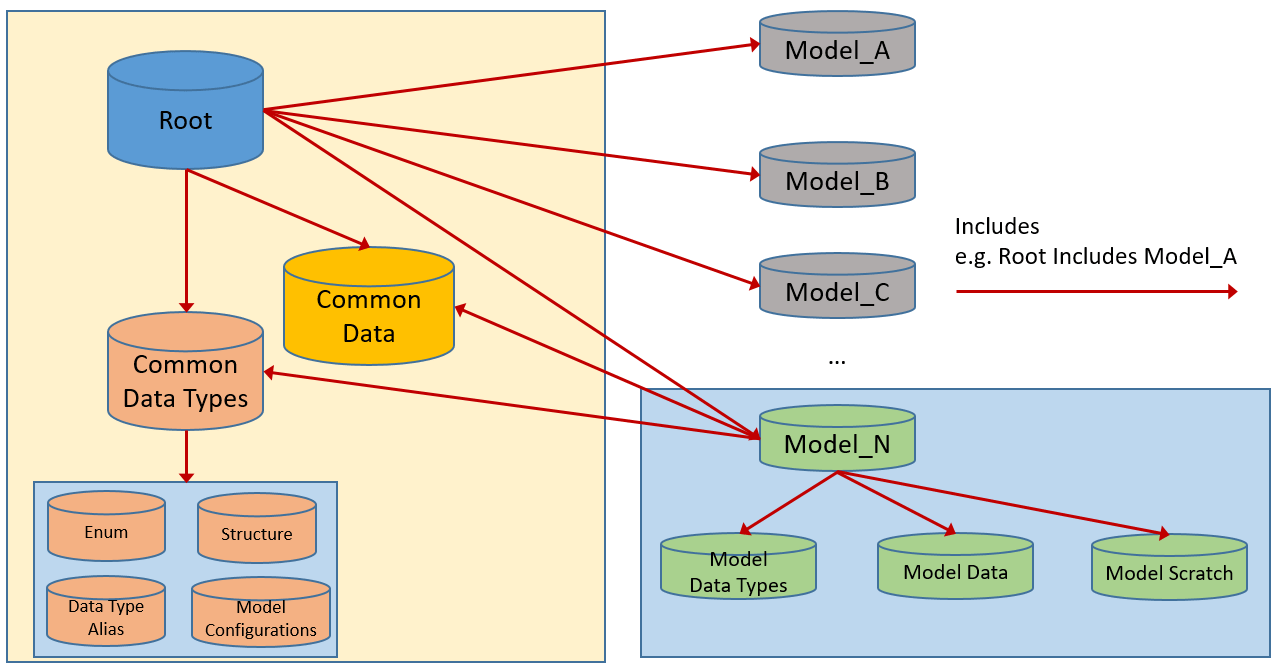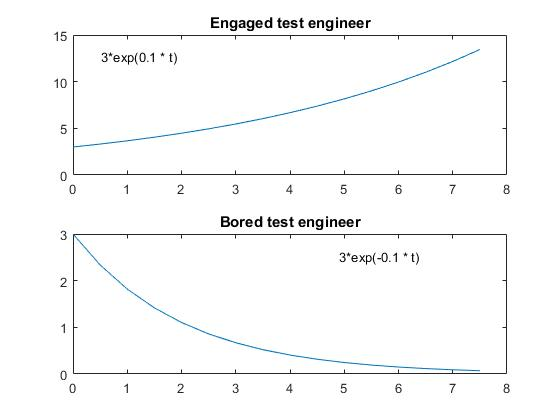## Model-Based Design Walk through: Part 4: Data

This post is the fourth in a series of 8 video blogs, walking through the fundamentals of Model-Based Design. When taken as a whole, these videos provide the foundation stones for understanding and implementing Model-Based Design workflows. I will be using a simple Home A/C system for my example; however the principals apply to everything from Active suspensions to Zonal control.(1)

With this post I cover the basics of data management, both for the model and configuration settings.

## Footnote

1. Stay in the zone, even when you zone out!
2. Take it with a grain of salt but how you pronounce the word “Data” may be dependent on ST-NG

## Managing data

In previous posts, I have covered data attributes and data usage.  In this post, I cover data management.  Within the Model-Based Design workflow, and traditional hand coding environments, there is a concept of model scoped and common data.  This blog post will use Simulink specific concepts for Data Dictionaries to show how scoped data can be achieved.

## What is in common?

Deciding what goesinto the common versus the model specific data dictionary is the primary question that needs to be asked at both the start of the project and throughout the model elaboration process.  There is always a temptation to “dump” data into the common data dictionary to “simplify” data access.  While in the short run it simplifies access, in the long run, doing so creates unmanageable data repositories.  So, again, the question is “what goes in there?”

### Common data type specificationThe common data types consist of four primary entries, each of which is created as a separate sub-dictionary.

• Structure definitions
• Enumerated data types
• Data type aliases
• Model configurations

In all 4 cases, these bits of information should be used in a global scope.  For example, structures used as an interface definition between two models or an enumerated data type that is used for modal control across multiple models.  In contrast, structures that are local to a single model should not be part of the common data types sub-dictionary.

### Common data

Like the common data types, the commondata consists of sub-dictionaries.  In this case, there are three.

• Physical constants
• Conversion factors
• Common parameters

The first two are simple to understand; instead of having the engineer put in 9.81 (m/s) for each instance of the force of acceleration a physical constant (accelGravMetric) can be defined.  Likewise, instead of hard coding 0.51444 you could have a parameter Knots_to_meter_p_sec.  (Note: in the first case, 9.81 is a value that most engineers would know off the top of their head.  The second case most people will not recognize and it results in “magic numbers” in the code.  This is compounded when people “compact” multiple conversion factors into a single conversion calculation and the information is lost)

The final sub-dictionary, common parameters, is the most difficult to scope.  Ideally, it should be limited to parameters that are used in more than one model; or more than one integration model.  To prevent the “mushroom growth” of data in the common parameter data dictionary regular pruning should be applied.

Pruning data is the process of examining entries in a data dictionary and determining if they are needed in the common data or in a model specific dictionary.  Within the Simulink environment, this can be accomplished using the model explorer or programmatically## Model and integration model data dictionaries

In the section on model architecture, we discussed the concept of “integration models.”  An integration model consists of multiple sub-models, which, in turn, may contain sub-models.The pattern for the integration model data dictionary mirrors the pattern that was shown in the initial diagram; the “twig” of the model tree references the branches, which in turn reference all the way back to the root.## Final thoughts

The use of scoped data dictionaries allows users to logically organize their data while minimizing the amount of work that individual contributors need to take to maintain the data.  This approach does not eliminate the need for data maintenance however it does provide tools to aid in the work.

## Understanding data usage in Model-Based Design Part II

In the last post we looked at the characteristics of data now we will at how those characteristics define and support the model.

## Data defines the model behavior

Let’s imagine a model “estimate time to debug software.”  The first thing we could consider is the following simple mathematical model

bugsFound =3 * exp(timeCoef * T) dT
where: T = [0 : 7.5] (half hour lunch break)
timeCoef = [-0.1 : 0.2]

And consider two parameter sets

• Bored test engineer: timeCoef = -0.1
• Bugs found in 7.5 hours: 15
• Engaged test engineer: timeCoef = 0.1
• Bugs found in 7.5 hours: 52With this simple example, we see that changes to data affect the results of the model (equation).  In the actual model, we see multiple parameters associated with the “engaged” and “bored” test engineers.

Parameter Comment
BugsPerLine Common
LinesPerHour Engaged > Bored
GramCoffeePerHour Engaged < Bored
TimeAllocatedToFindBugs Commom

In a system level model, comprised of multiple integration models, there are multiple parameter sets.

In this example, we have two top-level integration models, the controller, and the vehicle plant model.  Within those integration models, there are multiple parameter sets

• Engine
• number of cylinder
• 8 cylinder
• 6 cylinder
• 4 cylinder
• Throttle body
• Standard
• Turbocharger
• Supercharger
• Transmission
• ….
• Drive line
• …..

## Next steps

As this post shows the amount of data consumed by a set of models quickly grows in complexity.  In an upcoming post, we will look at best practices for data management.

## Understanding data usage in Model-Based Design Part I

What is data and how is it used in a model centered development process?  We start by talking about different types of data.

## Types of data

• Algorithmic data:  data that is used or created by the algorithms.
• Constants:  data values that are used in calculations that are fixed; for example, pi.
• Parameters: data values that are used in calculations but can be tuned, or c by the engineer; for example a gain value for a PID controller.
• Calculated values (signals): signals are the result of the calculations in the system
• State: state data is a special case of signal data, it is the calculated data from previous time steps• System specification data: data that configures the system
• Configuration:  Unique to Model Based Design configuration data specifies some of the base functionality of the models calculations.  This includes things such as integration methods, sample times, and code formatting.
• Instance specification: meta data that specifies which set of algorithmic data is used to instantiate a given instance of the model(1).
• Variant: data that configures which blocks of code execute; these are distinct from execution control as they are either compile time or start up controlled.  For example compiler #define options.
• Verification and validation data: data that is used or created by the models and system for testing.
• Input data:  inputs to the system used to drive the tests
• Expected outputs: results based on inputs and the test configuration
• Test configuration: data that configures the model (through selection of algorithmic and system specification data) for an instance of a given test.

## Data attributes

All data has attributes that define how that data is interpreted by the system(2).  Some modeling environment explicitly exposes all attributes (Simulink, UML) while others (C, C++)  require users to have an external database to associate attributes.

Let’s focus on the attributes that all modeling languages share in common for algorithmic data; they are

• Data type:  double, single, int, struct, enum…
• Dimension: scalar, vector, matrix…
• Storage class: Where the data is instantiatedIdeally, within the development workflow the attributes, such as minimum and maximum values, are used by verification and validation tools to ensure the correct behavior of the model.

## Next post

In the next post I will look at how the data is used to configure models and model behavior.

## Footnotes

(1) The initial values of the state data may be included in this configuration set.  Initial values for state data is a subset of the parameter data.
(2) The specific attributes for a given class of data will differ.